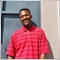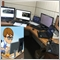# The Same stoploss and takeprofit but becomes different on the strategy tester5387

Hellow there ??

i have coded the EA That makes targets by pip value, it works perfect on USDCAD on testing but when testing sometimes the targets shrinks even though they display the same amount of points to positions sl and tp when i hover my mouse to them what might be the problem since they cause my EA to open too many trades in one candle the time that happens25836

Omega J Msigwa: what might be the problem5387

William Roeder:

what do you mean when you say broken code Sir162

Omega J Msigwa:

what do you mean when you say broken code Sir

Perhaps I didn't find your code when you raised your question.  If you point out a more specific scenario, highlight one example of an order you see different than what you expect, and share the code related to that scenario, I could help or make a suggestion.  The more specific you are in your question, the better chances to attract someone in the community to assist.25836

Omega J Msigwa: what do you mean when you say broken code Sir

Does it do what you want? If not, it is broken.

Do you really expect an answer? There are no mind readers here and our crystal balls are cracked.

Always post all relevant code.
How To Ask Questions The Smart Way. 2004

We can't see your broken code.5387

here is my code for opening positions
```void OpenPosition(const ENUM_POSITION_TYPE pos_type)
{
//--- check Freeze and Stops levels
/*
Type of order/position  |  Activation price  |  Check
------------------------|--------------------|--------------------------------------------
Sell Limit order        |  Bid                   |  OpenPrice-Bid  >= SYMBOL_TRADE_FREEZE_LEVEL
Sell Stop order            |  Bid                |  Bid-OpenPrice  >= SYMBOL_TRADE_FREEZE_LEVEL

Buying is done at the Ask price                 |  Selling is done at the Bid price
------------------------------------------------|----------------------------------
TakeProfit        >= Bid                        |  TakeProfit        <= Ask
StopLoss          <= Bid                          |  StopLoss          >= Ask
*/
if(!RefreshRates() || !m_symbol.Refresh())
return;
//--- FreezeLevel -> for pending order and modification
double freeze_level=m_symbol.FreezeLevel()*m_symbol.Point();
if(freeze_level==0.0)
freeze_level*=1.1;
//--- StopsLevel -> for TakeProfit and StopLoss
double stop_level=m_symbol.StopsLevel()*m_symbol.Point();
if(stop_level==0.0)
stop_level*=1.1;

if(freeze_level<=0.0 || stop_level<=0.0)
return;
//---
{
double sl=(InpStopLoss==0)?0.0:price-ExtStopLoss;
double tp=(InpTakeProfit==0)?0.0:price+ExtTakeProfit;
if(((sl!=0 && ExtStopLoss>=stop_level) || sl==0.0) && ((tp!=0 && ExtTakeProfit>=stop_level) || tp==0.0))
{
return;
}
}
if(pos_type==POSITION_TYPE_SELL)
{
double price=m_symbol.Bid();
double sl=(InpStopLoss==0)?0.0:price+ExtStopLoss;
double tp=(InpTakeProfit==0)?0.0:price-ExtTakeProfit;
if(((sl!=0 && ExtStopLoss>=stop_level) || sl==0.0) && ((tp!=0 && ExtTakeProfit>=stop_level) || tp==0.0))
{
OpenSell(sl,tp);
return;
}
}
}
//+------------------------------------------------------------------+
//+------------------------------------------------------------------+
{
sl=m_symbol.NormalizePrice(sl);
tp=m_symbol.NormalizePrice(tp);

double long_lot=InpLots;
//--- check volume before OrderSend to avoid "not enough money" error (CTrade)
double margin_check     = m_account.MarginCheck(m_symbol.Name(),ORDER_TYPE_SELL,long_lot,m_symbol.Bid());
if(free_margin_check>margin_check)
{
{
{
{
m_waiting_transaction=true;  // "true" -> it's forbidden to trade, we expect a transaction
}
}
else
{
{
m_waiting_transaction=true;  // "true" -> it's forbidden to trade, we expect a transaction
}
}
}
else
{
}
}
else
{
Print(__FUNCTION__,", ERROR: method CAccountInfo::FreeMarginCheck returned the value ",DoubleToString(free_margin_check,2));
return;
}
//---
}
//+------------------------------------------------------------------+
//| Open Sell position                                               |
//+------------------------------------------------------------------+
void OpenSell(double sl,double tp)
{
sl=m_symbol.NormalizePrice(sl);
tp=m_symbol.NormalizePrice(tp);

double short_lot=InpLots;
//--- check volume before OrderSend to avoid "not enough money" error (CTrade)
double free_margin_check= m_account.FreeMarginCheck(m_symbol.Name(),ORDER_TYPE_SELL,short_lot,m_symbol.Bid());
double margin_check     = m_account.MarginCheck(m_symbol.Name(),ORDER_TYPE_SELL,short_lot,m_symbol.Bid());
if(free_margin_check>margin_check)
{
{
{
{
m_waiting_transaction=true;  // "true" -> it's forbidden to trade, we expect a transaction
}
Print("#1 Sell -> false. Result Retcode: ",m_trade.ResultRetcode(),
}
else
{
{
m_waiting_transaction=true;  // "true" -> it's forbidden to trade, we expect a transaction
}
Print("#2 Sell -> true. Result Retcode: ",m_trade.ResultRetcode(),
}
}
else
{
Print("#3 Sell -> false. Result Retcode: ",m_trade.ResultRetcode(),
}
}
else
{
Print(__FUNCTION__,", ERROR: method CAccountInfo::FreeMarginCheck returned the value ",DoubleToString(free_margin_check,2));
return;
}
//---
}
//+----```

On the Strategy Tester

See the image i have attached ......Notice that sometimes it places wide targets (Stoploss and Takeprofit ) as i want it to be but in most of times these targets shrink eventhough they display the same pips amount .I have observed that this condition happens the most on Uptrend and downtrends where the EA opens a lot of trades since the targets is hit easily..This does not happen only to this EA but to many of them with different programming approach on MT4 and MT5 (it happens the most to my Grid EA's)  ...I am attaching the EA so that you can also see what might be the problem..

Files:25836

```      double price=m_symbol.Ask();
double sl=(InpStopLoss==0)?0.0:price-ExtStopLoss;
double tp=(InpTakeProfit==0)?0.0:price+ExtTakeProfit;
```

You buy at the Ask and sell at the Bid. Pending Buy Stop orders become market orders when hit and open at the Ask.

1. Your buy order's TP/SL (or Sell Stop's/Sell Limit's entry) are triggered when the Bid / OrderClosePrice reaches it. Using the Ask±n, makes your SL shorter and your TP longer, by the spread. Don't you want the specified amount used in either direction?